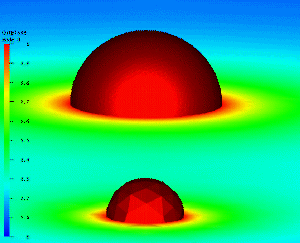Applications of FETK in General Relativity

The finite element code FETK has been used successfully to build adaptive numerical approximations of solutions to the nonlinear elliptic constraints in the Einstein equations. The domain has the structure of a Riemannian 3-manifold.

In the example below, GMV is used to visualize the solution produced by FETK. Each image is of the conformal factor; each is produced by separate solvers (with no communication!) as part of a new parallel algorithm for adaptive multilevel finite element methods.Here is another FETK solution of the constraints, in the presence of a single black hole. The left image is the conformal factor, and the right image is the first component of the W-vector. This solution was computed by Doug Arnold and Karen Camarda at Penn State using FETK.The paper describing the parallel algorithm used by FETK, which is joint work with Randy Bank, can be found here. A paper describing the application of FETK to the constraints in the Einstein equations, which is joint work with David Bernstein, will be available in the coming weeks. (Links to a number of examples from the paper can be found at the bottom of this page.) The constraint equations in the Einstein equations have the following form:Here is some related stuff: